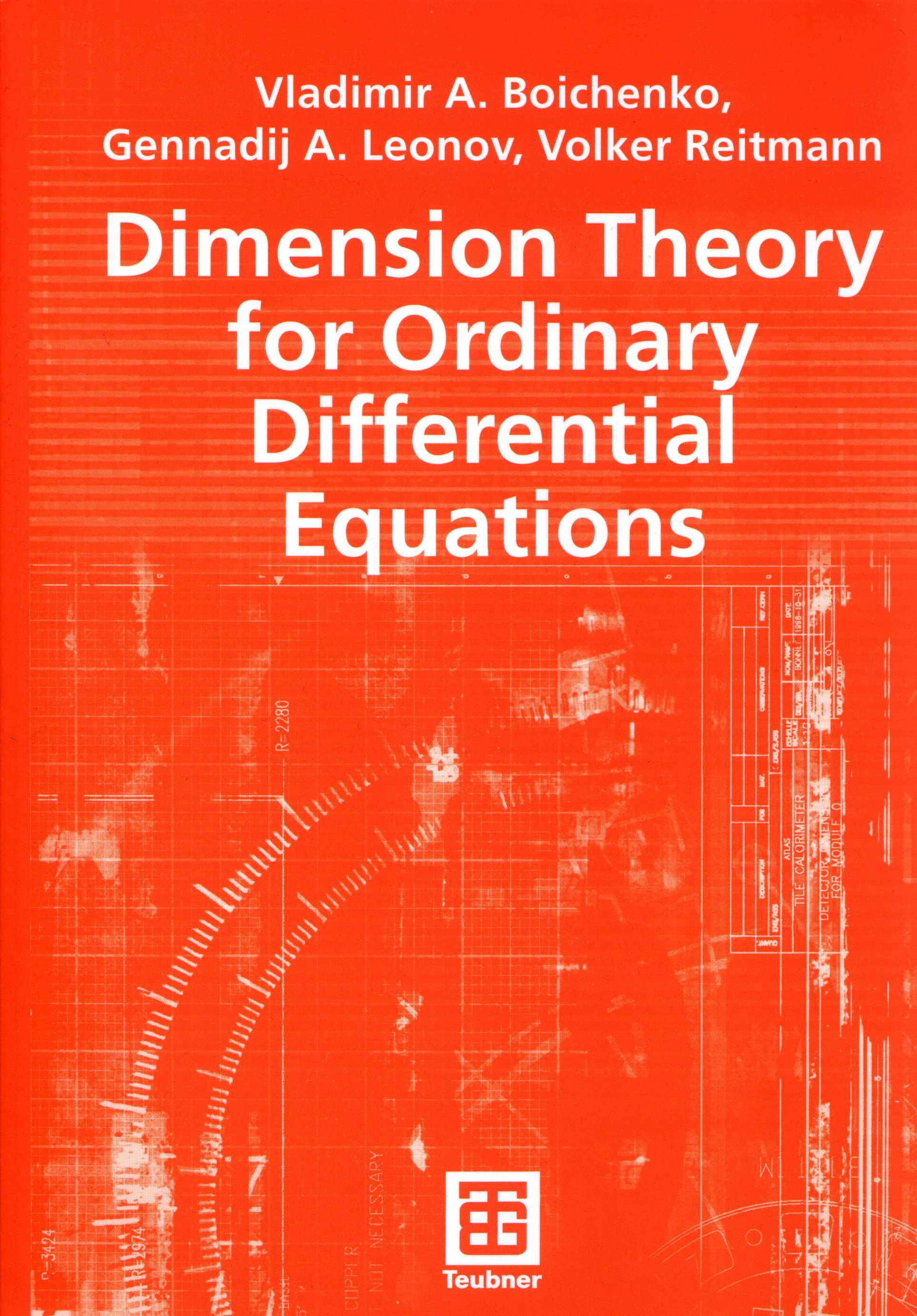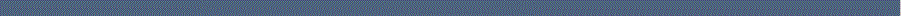Home Research interests Publications Conferences/Talks Supported projects since  2007 Supported projects  (1994 - 2006) Biographical Data

 Dimension Theory for Ordinary Differential EquationsBoichenko, V. A., Leonov, G. A. and Reitmann, V. Dimension Theory for Ordinary Differential Equations (English) B. G. Teubner Verlag / GWV Fachverlage GmbH, Wiesbaden, 441 p. (2005)

Contents

 I Singular values, exterior calculus and Lozinskii-norms 1 Singular values, exterior calculus and covering of ellipsoids 2 Singular value inequalities 3 Compound matrices 4 Logarithmic matrix norms 5 The Yakubovich-Kalman frequency theorem 6 Frequency-domain estimation of singular values 7 Exterior calculus in linear spaces II Attractors, stability and Lyapunov functions 1 Dynamical systems, limit sets and attractors 2 Dissipativity 3 Stability of motion 4 Existence of a homoclinic orbit in the Lorenz system 5 The generalized Lorenz system 6 Orbital stability for flows on manifolds III Introduction to dimension theory 1 Topological dimension 2 Hausdorff and fractal dimensions 3 Topological entropy 4 Dimension-like characteristics IV Dimension and Lyapunov functions 1 Estimation of the topological dimension 2 Upper estimates for the Hausdorff dimension 3 The application of the limit theorem to ODE's 4 Convergence in third-order nonlinear systems 5 Estimates of fractal dimension 6 Estimates of the topological entropy 7 Fractal dimension estimates 8 Upper Lyapunov dimension 9 Formulas for the Lyapunov dimension 10 Invariant sets of vector fields 11 Use of a tubular Carathéodory structure 12 The Lyapunov dimension as upper bound of the fractal dimension 13 Lower estimates of the dimension of B-attractors A Some tools A.1 Definition of a differentiable manifold A.2 Tangent space, tangent bundle and differential A.3 Tensor products, exterior products and tensor fields A.4 Riemannian manifolds A.5 Covariant derivative A.6 Vector fields A.7 Spaces of vector fields and maps A.8 Parallel transport, geodesics and exponential map A.9 Curvature and torsion A.10 Fiber bundles and distributions A.11 Recurrence and hyperbolicity in dynamical systems A.12 Homology theory A.13 Degree theory A.14 Simple d-linked parameterized m-boundaries A.15 Geometric measure theory A.16 Totally ordered sets A.17 Almost periodic functions Bibliography Index